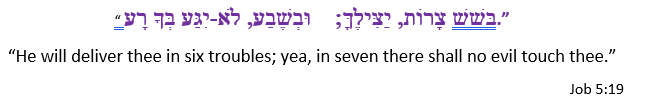Select Page

### The 4th Day of Creation

The clear mirror explains that on the 4th Day of Creation, the Creator established the 4 Cosmic Rings. Lights were set in the Heavenly sky to define the signs, festivals, days, and years.  Two large Lights, a greater Light and a smaller Light.

When G-d made the 4th Utterance, Vayomer Elohim (ויאמר־אלהים), He said “Let there be Lights in the Firmament (מְאֹרֹת בִּרְקִיעַ),” and He gave it the initials (מב), or 42, the initials used for all journeys in the Torah, as in the journeys these Lights travel around the Cosmic Wheels or Rings, and as in the journeys controlled by these Lights set in the Cosmic Rings. These journeys we refer to as time.

The word used for signs in this 4th paragraph of the Torah for the 4th Day of Creation in the 14th verse is “L’ottot (לאתת)” of gematria 831, the same as Alef (אלף), the first Name for the first Letter. Six (6) times that word was used on this 4th Day, a hint to the 24 hours in the Day that is defined in this 4th paragraph, as in the 6 Alefs (אלף) in the Torah’s first verse and 6 ellipses in the Cosmic Wheel, the Cosmic Ring of the Letters (לאתת).

The Heavenly Firmament (בִּרְקִיעַ הַשָּׁמָיִם) into which the Lights were set, has a numerical value of 777. While its 2 initials total 7, its final letters that total 110 hints to the convergence point of the 2 Primal Frequencies (1.1 and 27.5 Hz), or alternatively 670, which hints to the 670 paragraphs of the Torah and Binah (67) Concealed in the letters, its atbash value of the 2 words and 10 letters is 506, the uppermost of the 7 levels of the 42-Letter Name Matrix.

What was set into our Heavenly Firmament to shine on the central Earth on this 4th Day of Creation is the Cosmic Wheel of the Letters (לאתת); the Cosmic Wheel of the Festivals; the Cosmic Wheel of the Years; and the Cosmic Wheel of the Days—4 Cosmic Rings.  You probably should not proceed unless you have read at least Chapter 32, Spherical Time. If you are ready, a whole new world awaits. The Journey begins here if you missed it.

### The 4th Cosmic Ring

The days of the week are not part of a chain of time, as certain engines of confusion and illusion would have you believe; they are an independent cycle, a 4th Cosmic Ring until itself that interacts with the 3 others. It is a ring of 7 specific letters repeated every 7 Days, reflecting the Heavenly Firmament itself, every 168 or (42 x 4) hours. On every single day of the week 4 different letters yield their influence, the 4 Cosmic Addresses or 4 Loci of that specific cosmic 24-hour period within the 363 Day Cosmic Clock.

Every Day 4 different letter are activated. Every week, 4 letters x 7 Days are activated and in control, 28 in total/week, like the 28 Letters in the 7 words of Bereshit, the Torah’s first verse.

• In the Cosmic Wheel, where each letter represents a defined fraction of the 5 Elements of Creation, the letters change each and every day. This is in accordance with the specific Day in the specific order of the Triplets (14, 72, 27, 11, 15) that comprise the 5
• Another letter changes in the planetary Cosmic Wheel every lunar month/cycle, according to the plan of the 7 planets (5 plus the Sun and Moon) given to us by Abraham Avinu 3800 years ago.
• Another letter changes in the Precession Cosmic Wheel with every lunar month, which represents 1/12th of the precession, according to the 12 letters of the 12 constellations in the plan given to us by Abraham Avinu when G-d showed him the stars on the firmament.
• And yet another letter changes daily in cycles of 7 within the Cosmic Wheel of the Days of Creation, in accordance with the initials of the 7 sefirot (dimensions).

This is getting complicated, so we have the still silver pool recite this to us three times and we ask to see the 4 rings again and again until it sinks in and gets burned into our consciousness. Usually, the still small voice warns us when deep wisdom is about to be revealed.  I guess this time it figured we either saw it or we did not.

Man calls it astrology and entertains himself with it. The Inner Cosmos calls it existence.  Regardless of what we see when we look into the sky or think we see, without the Cosmic Rings that generate Zeir Anpin there is no sky, nor life, nor body, nor mind.

### The Day of Healing

The clear mirror explains that on the 5th Day of Creation, the Creator Uttered His first Blessing over the Earth. The numerical valued for “He Blessed (ויברך)” is 238 or 1/42. This was also the first day that there was no division within the Creation process. On the first 4 days we are specifically told about the division: light from darkness, water from water, land from sea, etc.

Thanks to the Cosmic Wheels every day of the week has a specific vibrational energy aligned with the 7 Days of Creation, and the 5th day of the week aligns with the 5th Day of the repeating wave of 7 Days.  Its vibrational energy is that of healing.

The Letter on the Cosmic Wheel that repeats on the 5th Day every 7 Days is the letter Hei (ה) of numerical value 5, the only Day that such an alignment occurs.

“The Fifth Day (יוֹם חֲמִישִׁי)” has a gematria katan of 28, as in the number of letters in the Torah’s first verse, and as in the (4 x 7) = 28 letters that guide us throughout every week/cycle of 7.

The value 28 is the balancing factor (power) around the central 14 of the Cube of Creation that makes every direction add up to 42. That central position of 14 with the Essential Cube is the value of the 6th and 7th letters (יד) of that 7 Day cycle. Together, the letters of the 5th, 6th and 7th Days spell “The Hand (היד),” as in the Hand of the Creator, reminding us of the 5 fingers on “The Hand(היד).” This also indicates that the vibrational energy of those 5th, 6th and 7th Days work in concert; after all, (5 + 6 + 7) = 18, life, and “The One.”

### The 4 Letters of Our DNA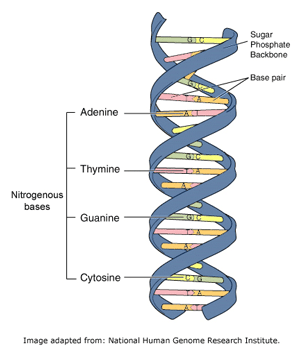More significantly, the numerical value of “The Fifth Day (יוֹם חֲמִישִׁי)” is 424, that of Moshiach Ben David, and of the 4 nucleotides (AGTC) of our DNA that add up to 424. There are 4 letters that guide the Cosmos through the 4 Rings of the Cosmic Wheel and 4 letters that guide life on Earth in the center of those Rings.

We are reminded that the 43 or 64 codons of our DNA correspond to the complete value (64) of the central position (50 + 14) of the Essential Cube of Creation, and that the two nucleotides (TC) correspond to the two letters Tav-Caf (תכ), the guiding letters of the 7 doubles, specifically of the Sun (כ) and the Moon (ת) in the Cosmic Planetary Wheel.

The 127 or (271) combinations of the 7 Words of the Torah’s first verse contain 64 null or counterspace cells, and it can be envisioned that they are filled by the 64 codons of our DNA. Then since the number 37, as in (37 x 73) = 2701, the value of that first verse, is at the heart of it in multiple ways, we find it fascinating that the ordinal value of the 4 nucleotides (AGTC) of our DNA is likewise 37.

And as if we are still having a hard time finding the hand of the Creator in our DNA, the still clear pool reveals that the 4 nucleotides (AGTC) when arranged in the sequence (GATC) are equivalent to 3142, as in the gematria katan (3142) of the 3-part Holy Name (אהיה־אשר־אהיה), and in the 3142 value of the two 3-fold expanded Holy Names YHVH (יהוה) and Ehyeh (אהיה).

The clear mirror then shows us that the sum total of the values of the 4 sets of Letters for all 363 Days divided by 3142 is 33.543 with 543 being the standard value of the Name (אהיה־אשר־אהיה).

We, our bodies, must physically be influenced by the vibration and the Letters of the Day of the weekly cycle more than we realize because the total value of the 7 controlling Letters for the 7 Day cycle is 480, and (48019), the value of the letters for the 5th, 6th and 7th Days (היד), is 461, which equals the complete value of our 4 nucleotides (AGTC): (424 + 37) = 461.

While some of us realize that there are 46 Chromosomal strands in our DNA, others make the Adam and Eve connection that Adam (45) + Eve (19) = 64 codons. In ancient times, people believed that vibration and frequency held the key to health, and more recently millions of people have come around to that same thinking. In between, that belief has been obscured and concealed to put it politely. We all know what we got instead. The Torah told us everything we needed to know.

### Shedding Light on the 5th day

The ordinal value in the two words “the Light (האוֹר)” duplicated and referred to in the phrase “Let there be light. And there was light. (יְהִי אוֹר; וַיְהִי-אוֹר),” and specifically again in the Torah’s 4th verse “וַיַּרְא אֱלֹהִים אֶת-הָאוֹר, כִּי-טוֹב; וַיַּבְדֵּל אֱלֹהִים, בֵּין הָאוֹר וּבֵין הַחֹשֶׁךְ” is 64. Their standard value is 424. This is the Light, the energy, and the vibration that empowers our DNA and that is especially available to us on the 5th Day, every weekly cycle.

That same sum of the 4 sets of Letters for all 363 Days when divided by 424 is 248.5708, as in the 248 dimensions and columns of the Torah and the year Israel became a nation, 5708 HC, 70 years before 5778, the Event Horizon. It is also 2485, the 70th Triangular Number, and 708, the 42 Letters of the Name YHVH (יהוה) hidden in 5 words of the 248-word Shema.

The value of the initials of “The Fifth Day (יוֹם חֲמִישִׁי)” spells Chai (חי), life, as in the value 18 of “The One (האחד)”, and of the 4 initials (יאוא) in the phrase “Let there be light… (יְהִי אוֹר; וַיְהִי-אוֹר),” in the Torah’s 3rd verse.

“The Fifth Day’s (יוֹם חֲמִישִׁי)” ordinal value is 91, the value of the YHVH-Adonai unification (יאהדוני) and the midpoint of the 5 portals of the Divine summation path (1 – 13 – 91 – 455 – 1820), the Path of One.

The value 91 is also the sum of the squares of the 6 Days (12 +22 + 32 + 42 + 52 + 62), meaning the channel is open through the YHVH-Adonai (יאהדוני) unification through all 6 dimensions of Zeir Anpin to refresh and rejuvenate our Life.

Meanwhile, the sum of the cubes of the 6 Days (13 +23 + 33 + 43 + 53 + 63) = 441 or 212, which is 42 less than 483, the sum of the 6 ordinal values for the 6 Days, which translates into the Truth plus the Source equals the Creation of the 6 Days, Zeir Anpin.  The Truth, of course, represents the Name of Binah, Ehyeh2 (אהיה)2.

### Shavuot

The sum of the cubes (13 +23 + 33 + 43 + 53 + 63 + 73) for all 7 Days of Creation and of the 7 Days of the week is 784 or 282, power (כח) squared, which is why spiritually the festival of weeks, Shavuot (שבועות), that occurs immediately after the 7 x 7 Omer cycle has a numerical value of 784.

Each of those 7 weeks represents an incremental climb to the top of the Tower of Truth, the last 7 cubes, each of those cubes and/or weeks represents a climb back to the Beginning, through the 7 words and 28 Letters of Creation, the Torah’s first verse.  It is also why the ordinal value of Shavuot (שבועות) is 73, as in the 73rd Triangular Number, 2701, the sum of the integers from 1 – 73, the numerical value of that first verse.  The distance between the 70th Triangular Number to the 73rd is 216, as in the 216 Letters in the 72 Triplets.

### The Menorah Redux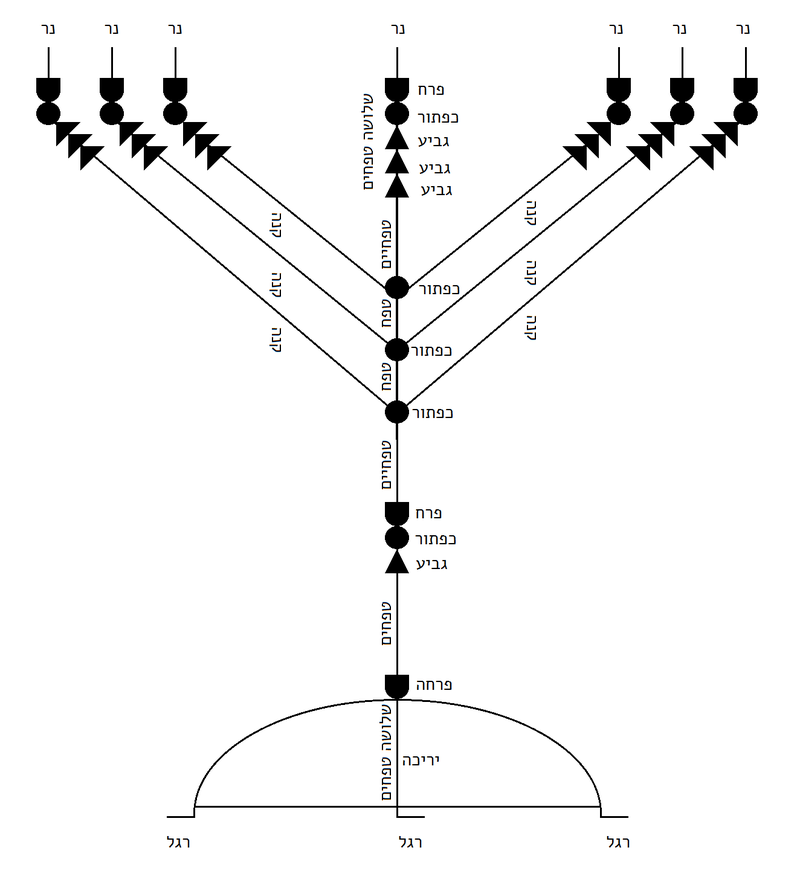In describing the Menorah, the 3 knobs in the center of the central candlestick must act as a bridge between the 6 arms (branches) in specific pairs, the 1 + 7 pair, the 2 + 6 pair, and the 3 + 5 pair, or 8, 8, 8 for 24, as in the 24 hours in each of the 6 Days. When the candlestick or 4th lamp is added, the 7 lamps equal (24 + 4) or 28, as in the 28 letters in the 7 words of Creation.

As noted previously, the Menorah of 42 elements and 7 Lamps is one of the 42 elements of the Mishkan and is akin to the 42-Letter Name Matrix of 7 levels, with the central candlestick equivalent to the central Path, the 4th level, of the 42-Letter Name Matrix.

The 3 legs (רגל) of the Menorah each have a numerical value of 233, which is known to the kabbalists as the sum of the 2nd iteration of both Ehyeh (אהיה) and the YHVH (יהוה) or (161 + 72). The 3 legs together have a numerical value with the kolel (3) of 702, that of Shabbat. The clear still pool explains that this 3-pronged base of the Menorah is aligned with the base of the 72 Names (Triplets) whose 9th or bottommost row has a numerical value of 702 and whose digits sum to 72.

When the 12 counterspace sections (טפחים) are added to the 12 spatial elements, the conjoined knobs, flowers, cups, with the 3 legs, the total is 27, as in the 27 positions/letters in the Essential Cube of Creation.

Of those 12 counterspace sections, 6 are in the central candlestick. When they are added to the 12 spatial elements of that same candlestick, the total of 18 aligns with “The One (האחד).”

### The Power of Healing

Every Rosh Chodesh or New Moon, 12 times a year, we read from parsha Pinchas, which the Zohar tells us is imbued with the power of healing. This is the 130th paragraph of the Book of Numbers, chapter 28. These few verses contain the word “The One (האחד)” 3 times.

The sum of the cubes for all 7 Days is 784 or 282, power (כח) squared, like the gematria katan (28) of the “The Fifth Day (יוֹם חֲמִישִׁי).” So, through its ordinal value of 91, the 5th Day forms a bridge to the 7th Day to Shavuot and to the Torah’s first verse, in the same way that the 5th Triplet in each of the 4 Sets of the Essential Triplets of Creation plus the One (345 + 57 + 25 + 46 + 1) = 474, Da’at.  Those 4 Triplets contain the one Triplet known to be used specifically for healing (מהש).

### Healing from Within the 72 Triplets

A little known gematria cipher, adds together the sum of the Triangular Numbers associated with the Hebrews letters, and when applied to Emet (אמת), Truth, it totals (1 + 91 + 253) = 345, the value for the 5th Triplet in the 72 Names (מהש) and of Moses (משה) and Hashem (השם). The power of healing through vibration.

When G-d made the 4th Utterance in verse 14, Vayomer Elohim (ויאמר־אלהים), He said “Let there be Lights in the Heavenly Firmament (מְאֹרֹת בִּרְקִיעַ הַשָּׁמַיִם) and He gave it the initials (מבה), of numerical value 47.

The secret of Power, koach (כח) is that its complete gematria is (28 + 19) = 47, which equals Ehyeh (אהיה) plus the YHVH (יהוה), the connection between Binah and Zeir Anpin. Within the 72 Names (Triplets), there are 4 which have the value 47: the heads of the 5th and 6th Candles, which are the 13th and 14th Triplets respectively (יזל) and (מבה); the 30th (אום); and the 55th (מבה).  The 14th and 55th Triplets (מבה) are the initials to the “Lights in the Heavenly Firmament (מְאֹרֹת בִּרְקִיעַ הַשָּׁמַיִם)” in the 14th verse of the Torah, whose final letters numerically spell Da’at (474). The complete value of (מבה), whether in the triplets or the initials is (47 + 20) = 67 (Binah).

The sum of the locations of the 4 Triplets within the 72 Triplet Matrix is (13 + 14 + 30 + 55) = 112, as in the full 112 Triplets, and the small gematria value of 3 of the Names are 425, 425, and 173, all resonating with the power of the 42-Letter Name connected with the 5th Day.

We are then reminded that the sum of the square roots of the 9 rows of the 72 Triplet Matrix is 280.0 as in 10 x 28 (כח), but also as in the 5 final letters (ךםןףץ) whose total is 280, and in the first two iterations of the Names Ehyeh (אהיה) and the YHVH (יהוה), (47 + 233) = 280.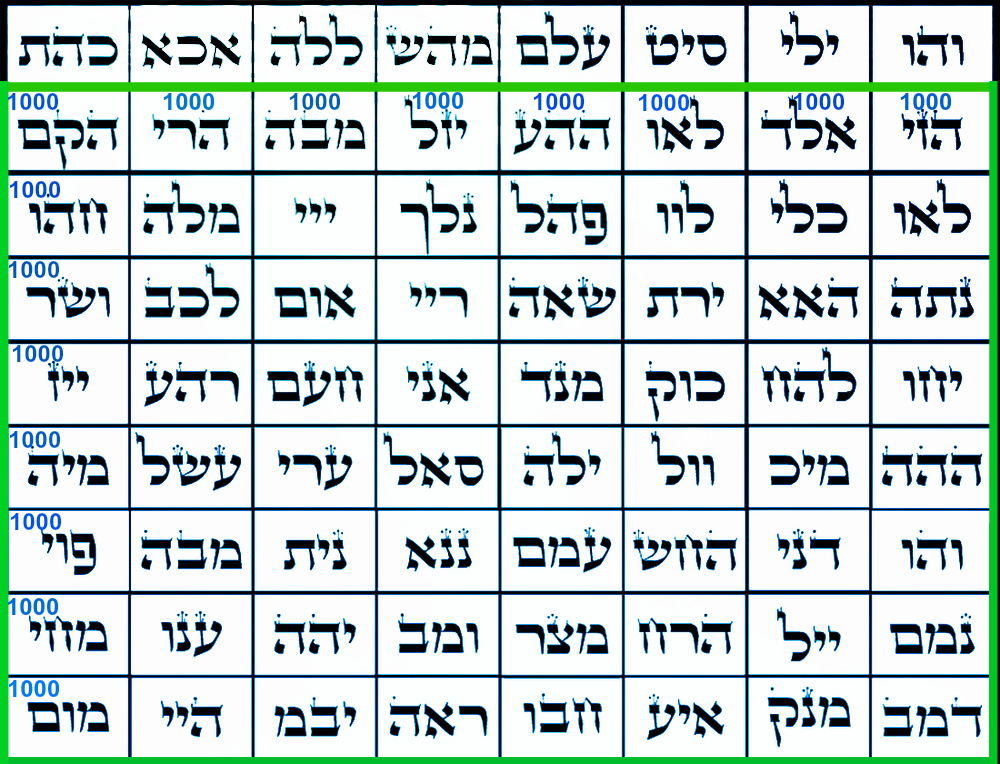We know the 72 Triplets are divided into 9 Candles which we light on the 8 Days of Chanukah and that the 9th Candle is the first row of the 72 Triplet Matrix, leaving the other 8 Candles to equal exactly 8000. Alternatively, the 8 subsequent rows also equal 8000, an average of 1000 per row, a reference to Binah. The clear mirror shows us the Matrix in standard gematria: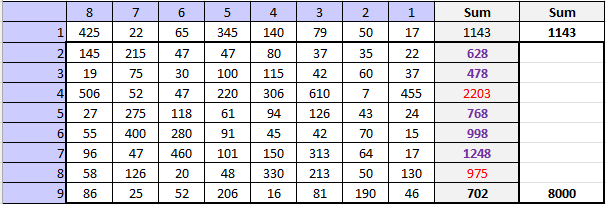The clear mirror wants us to note that there are 27 triple digit numbers, and 1 single digit one, as in 2701, leaving 44 double digit numbers, as in the 44 Chanukah candles lit over the 8 Days. Moreover, there are only 7 numeral 8’s out of the 170 total digits, yet like most of the Biblical dates that end in 8, five (5) of the rows’ sums end in 8.

We were already told that the final row totals 702, as in Shabbat (702) and we notice that the 2nd row totals twice (2) Pi (π), as in the formula of a circumference of a circle. What we miss until the clear mirror highlights it for us is that the sum of the other non-8 rows (2203 + 975) = 3178 together end in 8, and that 3178 HC is 160 years before the year 3338 HC, when the First Temple was destroyed. That is a bit of a stretch for us, until we are shown that (2203 + 975 + 702) = 3880, or 50 years from the destruction of the Second Holy Temple in 3830 HC, and that (160 + 50) = 210.

With a silvery splash, the clear mirror drops the mike.

We want to walk away, but it continues explaining that (80003880), in other words the 5 rows that end in 8, equals 4120. Now while 4120/8 = 515, a number that has just come up 3 times for us in rapid succession, the value 412 we know well as the Name of the letter Bet (ב), the first in the Torah, and as in 3 x the Phi(φ) angle, (3 x 137.5o) at the center of Spherical Time.

The clear mirror pool now shows us the 72 Name Matrix as a grid of 1 – 8 columns and 1 – 9 rows and multiplies each coordinate: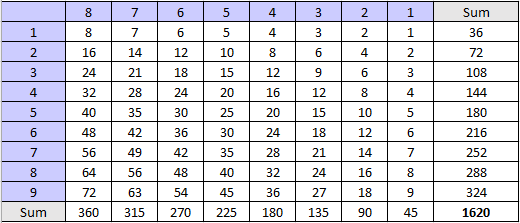Like the 9th or base row that matches the base of the Menorah in terms of 702 and 72, we see that since each of the digits in the 9th row sum to 9, the sum of the digits in this 9th row of the resultant grid of 72 is likewise 72, but it is the 1620 total that really stands out.

The clear mirror mentions that the 9 is a specific reference to the 9 Candles and that there are 4 addition sums of 9 within the grid of 72, making 13 in total, a specific reference to the 13 Candles in total. Furthermore, the sum total of 1620 equals 25042 with 250 being the value of ner (נר), candle, and thus 1620 is also 6480/4, the result of all those Torah element equations.

Moreover, it points out that each sum of the individual 8 columns and each sum of the individual 9 rows relates to a fraction of a circle (360o). All together, those 17 fractions equal 9 full circles. It is telling us this just in case we thought that all that went into the 72 Triplets was the wrapping of 3 consecutive verses of 72 letters, as opposed to layer upon layer of planning.

The whole circle and 72 Triplet Matrix connection reminds us that when 72 is the circumference of a circle, the diameter is (72/π), as in the Splitting of the Sea, and that it is equal to the Time Ratio (27.5/12).

### The Essential Cube of Creation and Time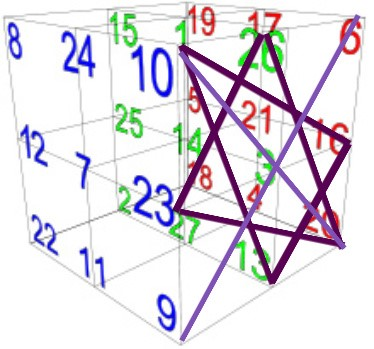Since that total value 1620 seems so familiar, besides the obvious 1000 (Binah) + 620 (Keter), the clear mirror reminds us that it is not only the product of the digits in the 58 words, letters, and verses in the Torah (3 x 9 x 6 x 2 x 5), but that it is also the product of the sofit value (999 x 5) of the 27 Letters of the Essential Cube of Creation (4 x 9 x 9 x 5), and that it is the complete value of the Names of the 6 Matriarchs of the Torah (Eve, Sarah, Rivka, Leah, Rachel, and Miriam). And speaking of the Essential Cube of Creation, 1620 is also the value of the 6 vertices of the Magen David of the East Face, an average of 270 per vertex.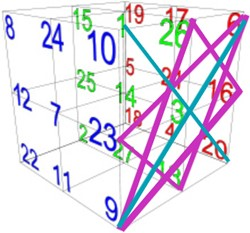Then demonstrating how immensely powerful the Essential Cube of Creation is, and alluding that we are overlooking its power in our daily lives, the clear mirrored pool shows us a different Magen David on the same face, and tells us that the sum of its 6 points (vertices) is 1656, the year of the Flood.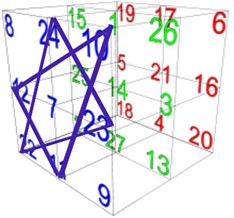As the cube slowly spins, we are shown the South face with the 9-11 in the corner. It has a Magen David whose 6 vertices equals 2001.

While the upper face has a Magen David whose 6 vertices and cross hairs equal 1776, as in the date of the Tower of Babel and the founding of the U.S.A.  Each face and each plane have their own set of Magen Davids and crosshairs, all symmetrically centered about position 14 (דוד), David, the center of Spherical Time.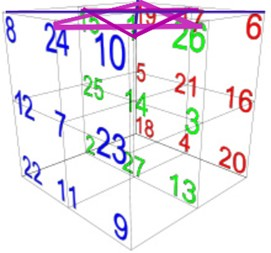The Inner Cosmos is giving us some of the most powerful tools in the universe, in existence, and it is wondering if we have elevated our conscious state enough to use to them.

The 72 Triplet grid returns to the surface of the silver mirror, and we are shown that the total value of the 72 Triplets with its kolel (216) is 9359 and that 9359/1620 = 5.777 and that the 2nd line/level of the 42-Letter Name is 729 or 93 and that 1620/729 = 2.222. The representations of the years (5777.8 + 222.2) = 6000, and of the equation (5.777 + 2.222) = 8.000, as in Binah and the 8000-value of the 8-row x 8-column square matrix is blatant.### The Fifth Day

The complete value of the “The Fifth Day (יוֹם חֲמִישִׁי)” is (424 + 91) = 515, as in the number of times Moses prayed to G-d to allow him to enter Israel and bring about Moshiach Consciousness, and as we have just learned, the value of the 5 rows that end in 8 divided by the 8 columns for an average of 4120/8 = 515. It is also notably the numerical value of Tefila (תפלה). Moreover, 515 is one less time than Moses needed in order to bring the final redemption, as in 516, the complete gematria of Da’at (דעת).  Moses knew that of course but was not allowed to deviate from the plan. Everything has its place and its purpose.

The 7 Days of the weekly cycle and of the 7 Days of Creation are designed to align with the 7 Levels of the 42-letter Name of G-d. The 5th Level has an ordinal value of 68, that of “Life, Chaim (חיים).” Both the 5th level and the word Life, Chaim (חיים)” begin with the letter Chet (ח) of numerical value 8, which the clear mirror connects to the 5 rows that end in the value 8. It then advises us that the sofit value of “Life, Chaim (חיים)” is 628, as in one of those 5 rows, the 2nd row, or twice (2) Pi (π).It further tells us that this 5th level begins with the Triplet (חקב) of numerical value 110, the harmonic convergence point for healing. Likewise, when the ordinal values of Da’at (דעת) or 42 is added to that of the 5th level, 68), we get (42 + 68) = 110, which we just saw in the final letters of the Heavenly Firmament (בִּרְקִיעַ הַשָּׁמָיִם) whereby 110 hints to the convergence point of the 2 Primal Frequencies (1.1 and 27.5 Hz). There can be no healing without a harmonization of the frequencies. It is done through vibrational resonance. When the 4 spiritual elements (earth, water, fire (light), air (firmament) are in harmony, as in (110/27.5) = 4, life returns to a state of balance. The 42-Letter Name is designed to balance life (68).

The ordinal value of Da’at (דעת) is 42, giving it a complete value of (474 + 42) = 516, as in that of the “The Fifth Day (יוֹם חֲמִישִׁי).”

Nonetheless, the numerical value of Da’at (דעת) though, when added to the value of the 6th level of the 42-Letter Name (474 + 230) equals the value of the 4th level, 704, the Path (דרך).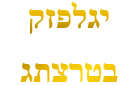The clear mirror informs us that this Path through the 4th level of the 42-Letter Name and through the 4th lamp, (the candlestick) of the Menorah, is the Path to the Tree-of-Life.  It brings up the matrix or magic square of 176 of the 16 physical elements and it takes us through a process whereby 176 is 4 x 44, then 8 x 22 and then that 704 becomes 4 x 8 x 22 or (32 x 22), representing the 32 Paths of Wisdom that is the Tree-of-Life and the 22 Letters/Names which are the connecting paths within those 32.

It then shows us the first level of the 42-Letter Name of numerical value 506, or (23 x 22).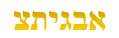That value, 506, as we well know, is the complete value of Moshiach Ben David and the sum of the first 11 squares, as in the 11 sefirot that include Da’at.

The clear mirror then adds that such is the power and design of the 42-Letter Name that its 1st and 4th levels work together as (506 + 704) to equal 1210 or (112 x 10), and since the 1st level is (23 x 22) and the 4th level is (32 x 22), together they are (55 x 22), representing 2 cubits for each of the 22 letters/Names or 2 cycles of the Primal frequency for each of them. This also explains why the entrance to the encasing pyramid is exactly 55’ off the ground, and the Gate of 42 to the Holy of Holies is 2-cubits thick.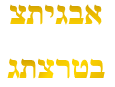And because this is also the Path of One, it explains the dynamic of (13 + 42) = 55.

### The Sixth Day

Opposite the Fifth Day on the Essential Cube of Creation is “The Sixth Day (יוֹם הַשִּׁשִּׁי)” whose numerical value is 671, as in Adonai (אדני) when filled in (נון־יוד אלף־דלת־) and as in the speed of light in mph (671 million mph). Its ordinal value is 86, as in Elohim (אלהים).

The 6th Day on the Essential Cube is the bottom face of the cube, at least from the singular perspective that we are viewing it. The clear mirror shows us the special dynamic of this face in that the center 3-part diagonal totals (9 + 900 + 90) = 999, and the 3 corner pieces on either side add up to (244 + 422) respectively or 666 for all 6. Their total of (999 + 666) is 1665, the complete numerical value of the 6 Patriarchs—Abraham, Isaac, Jacob, Joseph, Aaron, and Moses, like the complete value of the 6 Matriarchs that we just saw.

### The Seventh Day

Now, while “The Fifth Day (יוֹם חֲמִישִׁי)” is connected to 91 in the center of the Path of One (1 – 13 – 91455 – 1820), “On the Seventh Day (בַּיּוֹם הַשְּׁבִיעִי)” that is located in the center of the Essential Cube of Creation and closest to the Source has a standard value of 455, as in the 3 Aspects of the higher Name Ehyeh (אהיה).  The clear mirror first explains that there are 40 letters in the first 6 Days, as in the sum of the digits of the Path of One (1 – 13 – 91455 – 1820). Then it further explains that (91 + 455) = 546, the initials of the 10 sefirot (dimensions) of the Tree-of-Life, and that the complete value of the 10 letters in “On the Seventh Day (בַּיּוֹם הַשְּׁבִיעִי)” is 550, the sum of the 11 sefirot initials, which is purposefully designed to be (20 x 27.5 Hz).

It is also revealed that while the sum of all 5 connections in the Path of One (1 + 13 + 91 + 455 + 1820) = 2380 or 1/42, 238 is the numerical value of Rachel, the 5th of the 6 Matriarchs, and it equals (35 x 68), or the 5th of the 7 Days times Life (68).

The 7 Days and 7 aspects of the Essential Cube of Creation represent the lower 7 sefirot (Chesed, Gevurah, Tiferet, Netzach, Hod, Yesod, and Malchut) that comprise the 7 levels of the 42-Letter Name Matrix, leaving the 3 highest sefirot (Keter, Chochma, and Binah) dimensions beyond them. There is an 11th Dimension that forms the back of the Tree-of-Life and is associated with the 7th Day instead of Malchut, the world of illusion.  This is the mechanism through which everything on Shabbat, the 7th Day, is elevated. Everything done on Shabbat is on a higher level than we can achieve the rest of the week.  It is higher level frequency than the rest of the weekly cycle.  The letters corresponding to the of the 7 Days are (חגתנהיד), the initials of 7 sefirot (Chesed, Gevurah, Tiferet, Netzach, Hod, Yesod, and Da’at). Their complete value is 546, as in the value of all 10 initials of the 10 sefirot of the Tree-of-Life.

While the back letters (רהה) in the 3 highest sefirot total 210 or (5 x 42), the front letters (בינ-חכמ-כת) in the 3 highest sefirot (Keter, Chochma, and Binah), total 550, or exactly 20 cubits (20 x 27.5”), just like the value of “On the Seventh Day (בַּיּוֹם הַשְּׁבִיעִי);” and just like the initials in all 11 sefirot (כחבדחגתנהימ), whose first 5 initials (כחבדח) sum to 42; and just like the 4 final (תךץם) letters in “The Path of the Tree-of- Life (את־דרך־עץ־החיים)” that leads to the sefirot and also equal 550.

This is also just like the Gate of 42, the entrance to the 20-cubit per direction dimensions in the Holy of Holies 203 Cube within the Future Holy Temple.  The 100-cubit height of the Holy Temple that rises above the Holy of Holies matches the 11 initials (דצכעדשבאחמב) of the 10 plagues that sum to 541, Israel, and whose ordinal value totals 100, as in the 100th Prime Number, 541, and who’s last 2 initials (מב) of the final plague equal 42.

The clear mirror further reminds us of the complexity built into the 14 Triplets of the 42-Letter Name that the Essential Cube of 42 is based on, showing us that the 5th and 8th diagonally set Triplets of values (57 + 493) respectively also equal 550, while the 2 opposite Triplets, the 6th and 9th equal (330 + 211) = 541, Israel.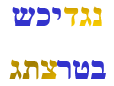While the product of (57 x 493) is 28,101, the sum of its 16 divisors is 43200, as in the two cubes of the Tree-of-Life and frequency of Binah (432 Hz), the product of (330 x 211)/3 = 23210, as in the 232-vlaue of the 4 aspects of the YHVH (יהוה), and the ubiquitous 210.

And as we learned a while back in the tower of the generations, the sum of the ages of the fathers on the dates that their lineage sons were born for the 11 generations from Shem to Isaac (100 + 35 + 30 + 34 + 30 + 32 + 30 + 29 + 70 + 100 + 60) total 550 years, which is 5 x 110, Joseph’s age, the convergence harmonic. It is also (50 x 11), an average of 1 Jubilee (50) year for each of them, and for each of the 11 sefirot, including Da’at, as in the central position of the Essential Cube of Creation. We are remined that this is also the average ordinal value (50) for each of the 15 steps of the Pesach Seder.

The gematria katan for “On the Seventh Day (בַּיּוֹם הַשְּׁבִיעִי)” is 32, as in the 32 Paths of the Tree-of-Life that we saw associated with the Path (22 x 32 = 704) and the 4th level of the 42-Letter Name (704). This also makes the total gematria katan for all 7 days equal to 173, as in the gematria katan for the 42-Letter Name of G-d that the Essential Cube of Creation is based upon, and that aligns spiritually and vibrationally with the 7 Days of Creation, and the weekly 7 Day cycle in the Cosmic Wheel. This is also why the Ashrei prayer that helps our other prayers bridge from one level to the next is comprised of 173 words and is structured on the 22 Letters/Paths and the 10 YHVH (sefirot) of the Tree-of-life. The one letter purposely left out is the letter Nun (נ) of numerical value 50 because of its elevation to Binah.

### Understanding Healing

The clear mirror then reiterates that “On the Seventh Day (בַּיּוֹם הַשְּׁבִיעִי)” that is located in the center of the Essential Cube and closest to the Source has a standard value of 455, as in the 3 Aspects of the higher Name Ehyeh (אהיה). It then adds that the 5th Triplet (מהש) of the 72 Names, which permutates to Moses (משה), has a numerical value of 345, but with a spelled-out backing of 110, as in nes, miracle, and as in the harmonic convergence, it has a gematria milui value of 455. We must understand that that backing is the same as the ordinal value (110) of “Let there be light. And there was light. (יְהִי אוֹר; וַיְהִי-אוֹר)” from the Torah’s 3rd verse.

This Triplet (מהש), which is likewise connected to the healing powers of “The Fifth Day (יוֹם חֲמִישִׁי),” is well known to be connected to the vibrations of “healing” and we can now understand that it has the inner light of healing. The energy of healing is freely accessible to us on both Days, the 5th and the 7th, as in (1 + 13 + 91 + 455 + 1820)/(5 x 7) = Life (68).

The still and clear mirrored pool also reminds us that another permutation of (מהש) is found in the 5th word of the Torah, H’Shamayim (השמים), alongside (ים) of numerical value 50 for the 50 letters in all 7 Days, and for the connection to the 50 Gates of Binah (H’Shamayim).

The clear mirror wants us to understand these bridges between the “The Fifth Day (יוֹם חֲמִישִׁי)” to the “On the Seventh Day (בַּיּוֹם הַשְּׁבִיעִי)” through the Divine summation Path (1 – 13 – 91455 – 1820) of One and through the powers for healing built into the 72 Triplets, the 14 Triplets of the 42-Letter Name, and through the Torah’s first verse within the 11 Triplets of Bereshit. While the complete value of the 4 initials (יחבה) in those 2 linked Days is 50, the complete value of the 14 final letters in the 14 words of all 7 Days is 499, as in Tz’va’ot (צבאות), as in the Lord of Hosts/Armies. The clear mirror adds that the ordinal value of Tz’va’ot (צבאות) is 49, as in 7 x 7 and the counting of the Omer and Jubilee. It further explains that (צב) has a complete value of 112 and “oht (אות)” means signs and/or letters. Lord of Hosts over the 112 Triplets.

Here is Wisdom. Protection and healing are accomplished through 7. The clear mirror shows us that the Path of One (1 + 13 + 91 + 455 + 1820) x 7 = 16660.